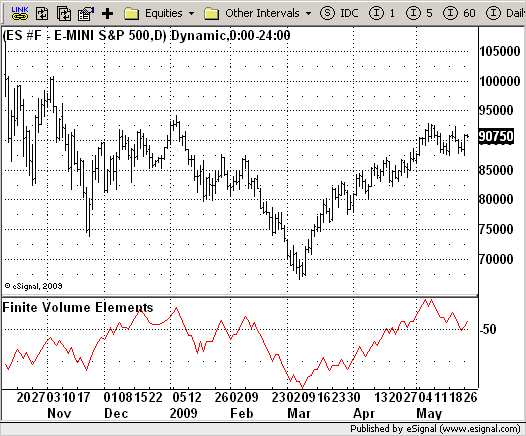# Finite Volume Elements (FVE)

ICE Data Services -

 FVE_Katsanos.efs EFSLibrary - Discussion Board

File Name: FVE_Katsanos.efs

Description:
Finite Volume Elements (FVE)

Formula Parameters:
Period : 22
Factor : 0.03

Notes:
The FVE is a pure volume indicator. Unlike most of the other indicators
(except OBV), price change doesn?t come into the equation for the FVE (price
is not multiplied by volume), but is only used to determine whether money is
flowing in or out of the stock. This is contrary to the current trend in the
design of modern money flow indicators. The author decided against a price-volume
indicator for the following reasons:
- A pure volume indicator has more power to contradict.
- The number of buyers or sellers (which is assessed by volume) will be the same,
regardless of the price fluctuation.
- Price-volume indicators tend to spike excessively at breakouts or breakdowns.`/*********************************Provided By: eSignal (Copyright c eSignal), a division of Interactive Data Corporation. 2009. All rights reserved. This sample eSignal Formula Script (EFS) is for educational purposes only and may be modified and saved under a new file name. eSignal is not responsible for the functionality once modified. eSignal reserves the right to modify and overwrite this EFS file with each new release.Description: Finite Volume Elements (FVE) Version: 1.0 05/25/2009 Formula Parameters: Default: Period 22 Factor 0.03 Notes: The FVE is a pure volume indicator. Unlike most of the other indicators (except OBV), price change doesn?t come into the equation for the FVE (price is not multiplied by volume), but is only used to determine whether money is flowing in or out of the stock. This is contrary to the current trend in the design of modern money flow indicators. The author decided against a price-volume indicator for the following reasons: - A pure volume indicator has more power to contradict. - The number of buyers or sellers (which is assessed by volume) will be the same, regardless of the price fluctuation. - Price-volume indicators tend to spike excessively at breakouts or breakdowns.**********************************/var fpArray = new Array();var bInit = false;function preMain() { setStudyTitle("Finite Volume Elements"); setCursorLabelName("FVI",0); setDefaultBarFgColor(Color.red,0); var x = 0; fpArray[x] = new FunctionParameter("Period", FunctionParameter.NUMBER); with(fpArray[x++]) { setLowerLimit(1); setDefault(22); } fpArray[x] = new FunctionParameter("Factor", FunctionParameter.NUMBER); with(fpArray[x++]) { setLowerLimit(0.01); setDefault(0.3); } }var xFVE = null;function main(Period, Factor) {var nBarState = getBarState();var nFVE = 0; if (nBarState == BARSTATE_ALLBARS) { if (Period == null) Period = 22; if (Factor == null) Factor = 0.3; } if (bInit == false) { xFVE = efsInternal("Calc_FVE", Period, Factor); bInit = true; } nFVE = xFVE.getValue(0); if (nFVE == null) return; return nFVE;}var bSecondInit = false;var xhl2 = null;var xhlc3 = null;var xClose = null;var xVolume = null;var xSMAV = null;function Calc_FVE(Period, Factor) {var nRes = 0;var nMF = 0;var nVlm = 0var nClose = 0;var nVolumeMA = 0;var nRef = ref(-1); if (bSecondInit == false) { xhl2 = hl2(); xhlc3 = hlc3(); xClose = close(); xVolume = volume(); xSMAV = sma(Period, xVolume); bSecondInit = true; } nVolumeMA = xSMAV.getValue(0) nClose = xClose.getValue(0); if (nVolumeMA == null) return; nMF = nClose - xhl2.getValue(0) + xhlc3.getValue(0) - xhlc3.getValue(-1); if(nMF > Factor * nClose / 100) nVlm = xVolume.getValue(0); else if(nMF < -Factor * nClose / 100) nVlm = - xVolume.getValue(0); else nVlm = 0; nRes = nRef + ((nVlm / nVolumeMA) / Period) * 100; return nRes;}`